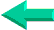This is an excerpt from Pierre Leclerc (www.excel-vba.com)

The 2 Most Important

Excel Functions AND
Formulas

SUMPRODUCT

AND

INDEX/MATCH

## Annex 2: Excel Spreadsheets  Date and Time Functions (21)

Note: The DATEDIF function does not show in the drop-down list of Excel functions (even in Excel 2007).

There are 21 functions in the "Date and Time" category.

 Functions What it Does DATE Returns the serial number of a particular date DATEDIF Calculates the interval in days, months or years between two dates DAY Converts a serial number to a day of the month HOUR Converts a serial number to an hour MINUTE Converts a serial number to a minute MONTH Converts a serial number to a month SECOND Converts a serial number to a second TIME Returns the serial number of a particular time TODAY Returns the serial number of today's date YEAR Converts a serial number to a year NOW Returns the serial number of the current date and time WEEKDAY Converts a serial number to a day of the week DATEVALUE Converts a date in the form of text to a serial number DAYS360 Calculates the number of days between two dates based on a 360-day year EDATE Returns the serial number of the date that is the indicated number of months before or after the start date EOMONTH Returns the serial number of the last day of the month before or after a specified number of months NETWORKDAYS Returns the number of whole workdays between two dates TIMEVALUE Converts a time in the form of text to a serial number WEEKNUM Converts a serial number to a number representing where the week falls numerically with a year WORKDAY Returns the serial number of the date before or after a specified number of workdays YEARFRAC Returns the year fraction representing the number of whole days between start_date and end_date

SUMPRODUCT  ¦  INDEX/MATCH  ¦  SUBTOTAL  ¦  ISERROR  ¦  HLOOKUP  ¦  LOOKUP  ¦  VLOOKUP
AVERAGEIF  ¦  AVERAGEIFS ¦  COUNTIF  ¦  COUNTIFS  ¦  SUMIF  ¦  SUMIFS  ¦  IFERRORBack home